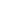Flat 50% off

Ends in

•• 11th
•• Physics
• Back to Doubt Clearing

##A particle is projected from a pipe at an angle $\theta = 45^o$ wtih the vertical at a point P with a speed $u = 10 ms^{-1}$. A strong horizontal wind gives a constant horizontal acceleration equal to $5 ms^{-2}$ to the particle such that the particle reaches the ground at point Q which is exactly below P. The height of point P (in m) from the ground is $[g = 10 ms^{-2}]$ (a) 30(b) 40(c) 50(d) 60

Hint

<p>&nbsp;</p> <p>&nbsp;</p>